# GRE Quantitative Reasoning: Coordinate Geometry Chapter Exam

Exam Instructions:

Choose your answers to the questions and click 'Next' to see the next set of questions. You can skip questions if you would like and come back to them later with the yellow "Go To First Skipped Question" button. When you have completed the practice exam, a green submit button will appear. Click it to see your results. Good luck!

### Page 1

#### Question 1 1. Estimate the coordinates of the midpoint of the line segment below: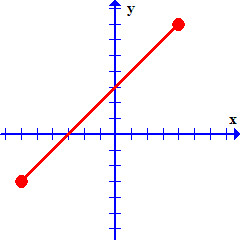#### Question 2 2. What is the slope of the line tangent to the curve given by the equation y = (x-1)²+2 at the point (1,2)?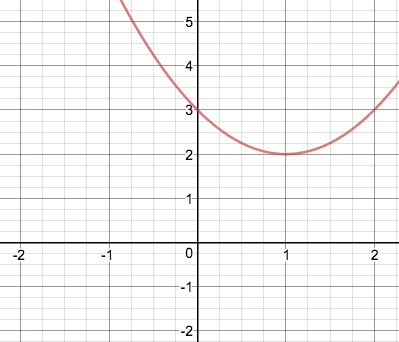#### Question 3 3. Which equation matches the graph below?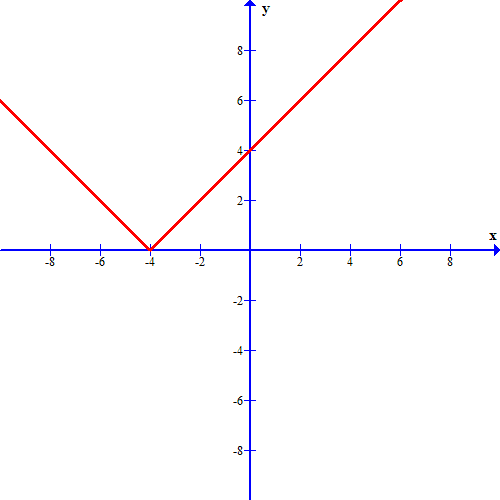#### Question 5 5. Which line is the transversal?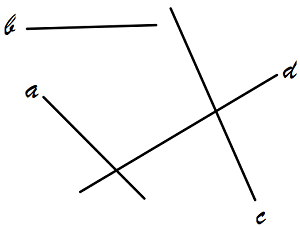### Page 2

#### Question 8 8. Which equation describes the following graph?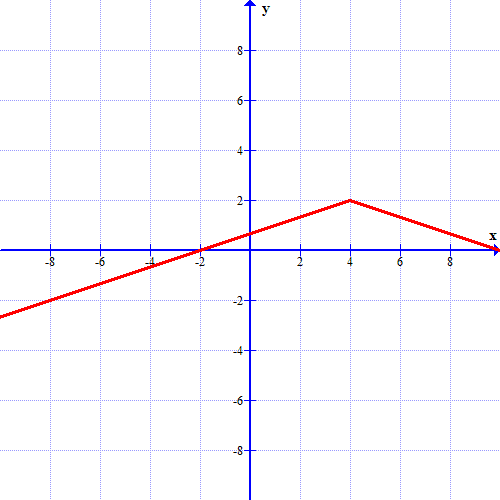#### Question 10 10. Which graph describes the following equation?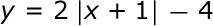### Page 3

#### Question 14 14. Which one of the following equations could work for the following graph?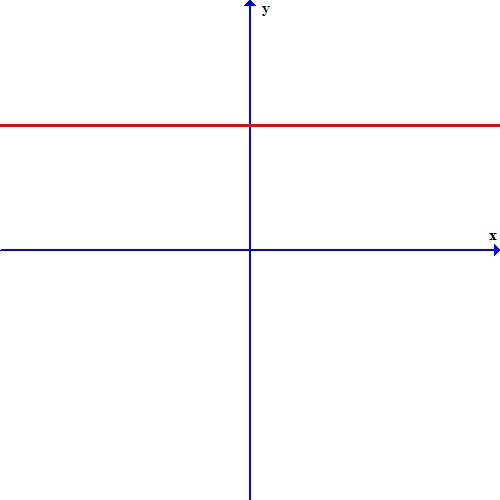### Page 5

#### Question 22 22. Which kind of transformation would change the purple function below into the yellow one?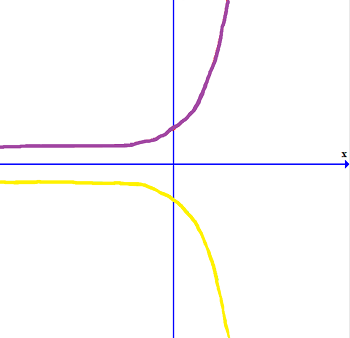#### Question 23 23. Which two lines are parallel?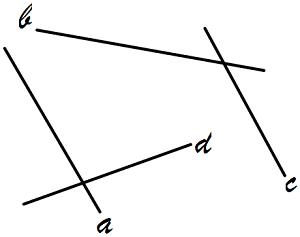### Page 6

#### Question 28 28. In the figure shown below, the graph of the yellow function is a translation of the graph of the blue function. If the expression for the blue function is y(x) = b(x), which of the following is the equation of the yellow function?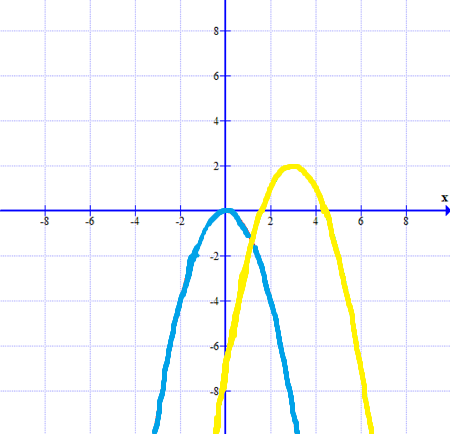#### GRE Quantitative Reasoning: Coordinate Geometry Chapter Exam Instructions

Choose your answers to the questions and click 'Next' to see the next set of questions. You can skip questions if you would like and come back to them later with the yellow "Go To First Skipped Question" button. When you have completed the practice exam, a green submit button will appear. Click it to see your results. Good luck!

Support